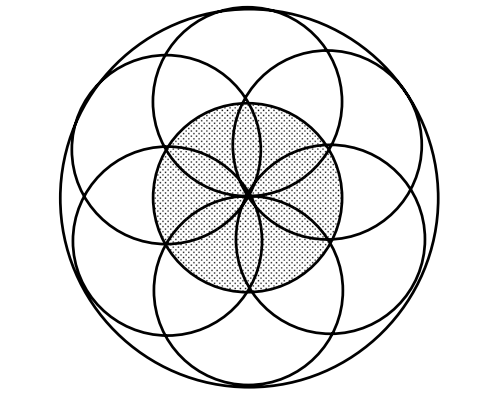Subject:
Mathematics

Topics: GeometryCircles
Common Core State Standard: K.G.1, 7.G.4,
Concepts:

· Diameter

Lesson:

Procedure: The objective of this activity is simply to get students thinking about circles and bringing to mind their prior knowledge of this topic.

There are eight circles in the figure.

In comparing the smaller circle to the larger circle, students’ initial response is likely to be simply that the larger circle is “bigger”. This is a good opportunity to point out that one of the reasons mathematics is valuable is that it provides a way to describe relationships exactly. How much bigger is the bigger circle? This should lead naturally to the question of “how do we measure the size of a circle?”, which in turn motivates the concepts of “diameter” and “radius”. (You may also choose to discuss “circumference” in this same context).

Note that “diameter” and “radius” each have two definitions: a specified line segment (as in, “draw a radius for this circle”), or the length of that segment (as in, “the radius is 5 centimeters”). Students will benefit from understanding both definitions and some practice deciding which definition is meant in various example statements.

### Looking for Circles1. How many circles can you find?

2. Trace one of the small circles and the large circle. How do they compare to each other?

Words:

Diameter: The length of a line segment that starts at one point on a circle, goes through the center, and ends at another point on the circle. This length is always the same, no matter which points on the circle are chosen. Sometimes the line segment itself is also called a diameter.

Radius: The length of a line segment from the center of a circle to any point on the circle. This length is always the same, no matter which point on the circle is chosen. Sometimes the line segment itself is also called a radius.